# Approximate differentiability

(diff) ← Older revision | Latest revision (diff) | Newer revision → (diff)

A generalization of the concept of differentiability obtained by replacing the ordinary limit by an approximate limit. A real-valued function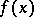of a real variable is called approximately differentiable at a point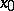if there exists a numbersuch thatThe magnitude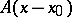is called the approximate differential ofat. A functionis approximately differentiable at a pointif and only if it has an approximate derivativeat this point. Approximate differentiability of real functions ofreal variables is defined in a similar manner. For example, for,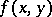is called approximately differentiable at a point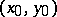if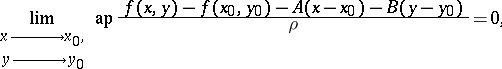whereandare certain given numbers and. The expression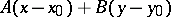is called the approximate differential ofat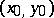.

Stepanov's theorem: A real-valued measurable functionon a setis approximately differentiable almost-everywhere on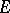if and only if it has finite approximate partial derivatives with respect toand toalmost-everywhere on; these partial derivatives almost-everywhere oncoincide with the coefficientsand, respectively, of the approximate differential.

The concept of approximate differentiability can also be extended to vector functions of one or more real variables.